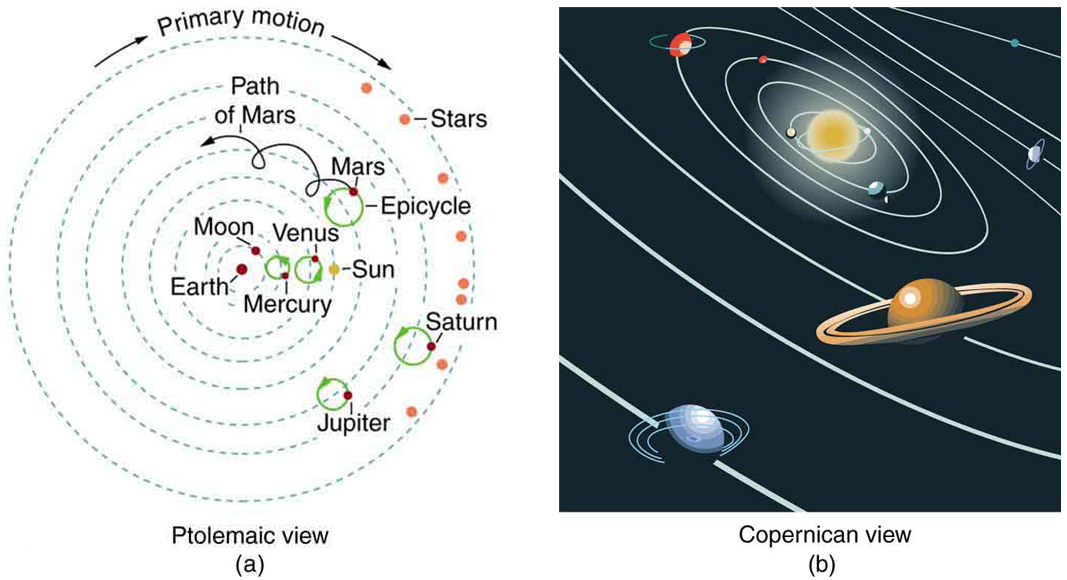# 6.6 Satellites and kepler’s laws: an argument for simplicity  (Page 4/5)

 Page 4 / 5

A non-satellite body fulfilling only the first two of the above criteria is classified as “dwarf planet.”

In 2006, Pluto was demoted to a ‘dwarf planet’ after scientists revised their definition of what constitutes a “true” planet.

Orbital data and kepler’s third law
Parent Satellite Average orbital radius r (km) Period T(y) r 3 / T 2 (km 3 / y 2 )
Earth Moon $3.84×{\text{10}}^{5}$ 0.07481 $1\text{.}\text{01}×{\text{10}}^{\text{19}}$
Sun Mercury $5\text{.}\text{79}×{\text{10}}^{7}$ 0.2409 $3\text{.}\text{34}×{\text{10}}^{\text{24}}$
Venus $1\text{.}\text{082}×{\text{10}}^{8}$ 0.6150 $3\text{.}\text{35}×{\text{10}}^{\text{24}}$
Earth $1\text{.}\text{496}×{\text{10}}^{8}$ 1.000 $3\text{.}\text{35}×{\text{10}}^{\text{24}}$
Mars $2\text{.}\text{279}×{\text{10}}^{8}$ 1.881 $3\text{.}\text{35}×{\text{10}}^{\text{24}}$
Jupiter $7\text{.}\text{783}×{\text{10}}^{8}$ 11.86 $3\text{.}\text{35}×{\text{10}}^{\text{24}}$
Saturn $1\text{.}\text{427}×{\text{10}}^{9}$ 29.46 $3\text{.}\text{35}×{\text{10}}^{\text{24}}$
Neptune $4\text{.}\text{497}×{\text{10}}^{9}$ 164.8 $3\text{.}\text{35}×{\text{10}}^{\text{24}}$
Pluto $5\text{.}\text{90}×{\text{10}}^{9}$ 248.3 $3\text{.}\text{33}×{\text{10}}^{\text{24}}$
Jupiter Io $4\text{.}\text{22}×{\text{10}}^{5}$ 0.00485 (1.77 d) $3\text{.}\text{19}×{\text{10}}^{\text{21}}$
Europa $6\text{.}\text{71}×{\text{10}}^{5}$ 0.00972 (3.55 d) $3\text{.}\text{20}×{\text{10}}^{\text{21}}$
Ganymede $1\text{.}\text{07}×{\text{10}}^{6}$ 0.0196 (7.16 d) $3\text{.}\text{19}×{\text{10}}^{\text{21}}$
Callisto $1\text{.}\text{88}×{\text{10}}^{6}$ 0.0457 (16.19 d) $3\text{.}\text{20}×{\text{10}}^{\text{21}}$

The universal law of gravitation is a good example of a physical principle that is very broadly applicable. That single equation for the gravitational force describes all situations in which gravity acts. It gives a cause for a vast number of effects, such as the orbits of the planets and moons in the solar system. It epitomizes the underlying unity and simplicity of physics.

Before the discoveries of Kepler, Copernicus, Galileo, Newton, and others, the solar system was thought to revolve around Earth as shown in [link] (a). This is called the Ptolemaic view, for the Greek philosopher who lived in the second century AD. This model is characterized by a list of facts for the motions of planets with no cause and effect explanation. There tended to be a different rule for each heavenly body and a general lack of simplicity.

[link] (b) represents the modern or Copernican model. In this model, a small set of rules and a single underlying force explain not only all motions in the solar system, but all other situations involving gravity. The breadth and simplicity of the laws of physics are compelling. As our knowledge of nature has grown, the basic simplicity of its laws has become ever more evident.(a) The Ptolemaic model of the universe has Earth at the center with the Moon, the planets, the Sun, and the stars revolving about it in complex superpositions of circular paths. This geocentric model, which can be made progressively more accurate by adding more circles, is purely descriptive, containing no hints as to what are the causes of these motions. (b) The Copernican model has the Sun at the center of the solar system. It is fully explained by a small number of laws of physics, including Newton’s universal law of gravitation.

## Section summary

• Kepler’s laws are stated for a small mass $m$ orbiting a larger mass $M$ in near-isolation. Kepler’s laws of planetary motion are then as follows:

Kepler’s first law

The orbit of each planet about the Sun is an ellipse with the Sun at one focus.

Kepler’s second law

Each planet moves so that an imaginary line drawn from the Sun to the planet sweeps out equal areas in equal times.

Kepler’s third law

The ratio of the squares of the periods of any two planets about the Sun is equal to the ratio of the cubes of their average distances from the Sun:

where $T$ is the period (time for one orbit) and $r$ is the average radius of the orbit.

• The period and radius of a satellite’s orbit about a larger body $M$ are related by
${T}^{2}=\frac{{4\pi }^{2}}{\text{GM}}{r}^{3}$

or

$\frac{{r}^{3}}{{T}^{2}}=\frac{G}{{4\pi }^{2}}M\text{.}$

does the force in a system result in the energy transfer?
full meaning of GPS system
how to prove that Newton's law of universal gravitation F = GmM ______ R²
sir dose it apply to the human system
prove that the centrimental force Fc= M1V² _________ r
prove that centripetal force Fc = MV² ______ r
Kaka
how lesers can transmit information
griffts bridge derivative
below me
please explain; when a glass rod is rubbed with silk, it becomes positive and the silk becomes negative- yet both attracts dust. does dust have third types of charge that is attracted to both positive and negative
what is a conductor
Timothy
hello
Timothy
below me
why below you
Timothy
no....I said below me ...... nothing below .....ok?
dust particles contains both positive and negative charge particles
Mbutene
corona charge can verify
Stephen
when pressure increases the temperature remain what?
remains the temperature
betuel
what is frequency
define precision briefly
CT scanners do not detect details smaller than about 0.5 mm. Is this limitation due to the wavelength of x rays? Explain.
hope this helps
what's critical angle
The Critical Angle Derivation So the critical angle is defined as the angle of incidence that provides an angle of refraction of 90-degrees. Make particular note that the critical angle is an angle of incidence value. For the water-air boundary, the critical angle is 48.6-degrees.
okay whatever
Chidalu
pls who can give the definition of relative density?
Temiloluwa
the ratio of the density of a substance to the density of a standard, usually water for a liquid or solid, and air for a gas.
Chidalu
What is momentum
mass ×velocity
Chidalu
it is the product of mass ×velocity of an object
Chidalu
how do I highlight a sentence]p? I select the sentence but get options like copy or web search but no highlight. tks. src
then you can edit your work anyway you wantByByBy OpenStaxBy Dindin SecretoBy Anonymous UserBy RhodesBy OpenStaxBy Ellie BanfieldBy OpenStaxBy Eddie UnverzagtBy Kevin MoquinBy Rhodes